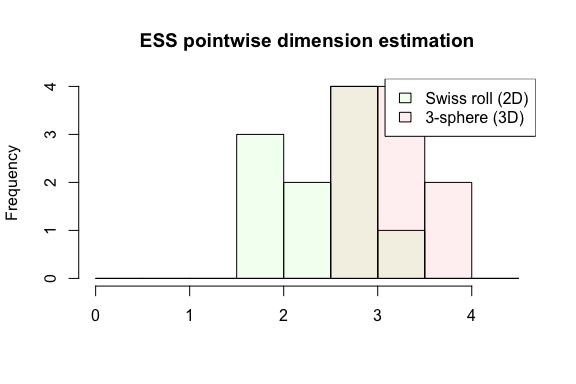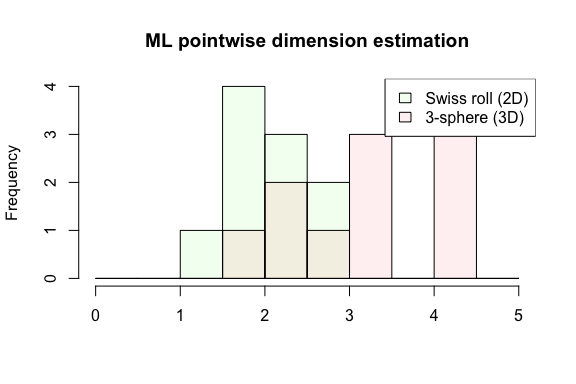# Overview of intrinsicDimension package

#### 2019-06-07

The intrinsic dimension of a data set is a measure of its complexity. Data sets that can be accurately described with a few parameters have low intrinsic dimension. It is expected that the performance of many machine learning algorithms is dependent on the intrinsic dimension of the data. Is has also been proposed to use estimates of intrinsic dimension for applications such as network anomaly detection and image analysis.

This package contains implementations of a variety of approaches for intrinsic dimension estimation: modeling distances by for example Maximum Likelihood, approximating hyperplanes using Principal Component Analysis (PCA) and modeling angular information and concentration of measure (ESS and DANCo methods). Ground truth data, i.e. data with known intrinsic dimension, can be generated with a number of functions modeling manifolds. The manifold dimension is the intrinsic dimension if the data is a manifold, otherwise the intrinsic dimension is usually defined as the Hausdorff dimension (which is a general measure of dimension that also applies to fractals.)

The package distinguishes between local, global and pointwise estimators of intrinsic dimension. Local estimators estimate dimension of a local data set, for example a neighborhood from a larger data set. For this estimate to be accurate the noise and the curvature of the data has to be small relative to the neighborhood diameter. A global estimator takes the entire data set and returns one estimate of intrinsic dimension. Global estimators has the potential to handle higher noise and curvature levels than local estimators, but require that the entire data set has the same intrinsic dimension. Pointwise estimators are essentially local estimators applied neighborhoods around each point in the data set, but sometimes information beyond the neighborhood is used, as in PCA with Optimally Topolgy Perserving Maps. Any local estimator can be converted into a pointwise estimator.

## Methods for estimating intrinsic dimension

• essLocalDimEst: ESS - Exploiting distribution of angles, Johnsson et al. (2015).
• dancoDimEst: DANCo - Exploiting distributions of angles and distances,Ceruti et al. (2012).
• pcaLocalDimEst, pcaOtpmPointwiseDimEst: Local PCA - Fitting approximate hyper planes through Principal Components Analysis. Fukunaga and Olsen (1971), Bruske and Sommer (1998), Fan et al. (2010).
• maxLikLocalDimEst, maxLikPointwiseDimEst, maxLikGlobalDimEst: Maximum Likelihood - Modeling distances, without noise (Hill, Levina and Bickel) or with noise (Haro), Hill (1975), Levina and Bickel (2005), Haro et al. (2008):
• knnDimEst: kNN - Exploiting distribution of distances, Carter et al (2010)

### Applying local estimators to non-local data sets

• asPointwiseEstimator: Converting local estimators to pointwise estimators:
• neighborhoods: Generating local data sets from data:

## Data distributions

### Local data sets (pieces of manifolds)

• hyperBall: Uniform distribution over hyper ball. This is the ideal ground truth distribution for local estimators.
• cutHyperPlane, cutHyperSphere: Piece of hyper plane or hyper sphere overlaid with noise. Note the noise is added before the piece is cut out, which is not the same as adding the noise afterwards. These can be used for evaluating how sensitive local estimators are to noise and curvature.

### Uniform distributions on manifolds

• hyperSphere: Uniform distribution over hyper sphere.
• hyperCube, hyperCubeFaces, hyperCubeEdges: Uniform distribution over hyper cube, over its faces or over its edges (strictly speeking the edges is not a manifold, but union of one-dimensional manifolds).
• swissRoll, swissRoll3Sph: Uniform distribution over swiss roll and swiss roll with 3-sphere inside.
• cornerPlane: Uniform distribution over a bent plane (2D).

### Non-uniform distributions on manifolds

• isotropicNormal: Isotropic normal distribution.
• oblongNormal: Non-isotropic normal distribution.
• twinPeaks, hyperTwinPeaks: Twin Peaks distribution (2D) and higher-dimensional versions, i.e. a valley and a peak in each dimension obtained from a product of sine functions.
• mHeinManifold, m14Manifold, m15Manifold: High-dimensional manifolds used by Hein and Audibert (2005) and Rozza (2012).

## Examples

library(intrinsicDimension)
## Loading required package: yaImpute

Local estimators applied to a simulated local data set with 30 data points, intrinsic dimension 50, noise dimension 100 and noise standard deviation 0.01.

local.data <- cutHyperPlane(Ns = 30, d = 50, n = 100, sd = 0.01)
essLocalDimEst(local.data, ver = 'a')
## Dimension estimate: 49.80429
## Additional data: ess
maxLikLocalDimEst(local.data)
## Dimension estimate: 20.14474
maxLikLocalDimEst(local.data, dnoise='dnoiseGaussH', sigma=0.01, n=100)
## Dimension estimate: 20.12549
pcaLocalDimEst(local.data, ver = 'FO')
## Dimension estimate: 28
## Additional data: gap.size

Global and pointwise estimators applied to data on a manifold with intrinsic dimension 2.

manifold.data <- swissRoll(500)
maxLikGlobalDimEst(manifold.data, k=10, unbiased = TRUE)
## Dimension estimate: 1.796268
maxLikGlobalDimEst(manifold.data, k=10, unbiased = TRUE, neighborhood.aggregation = 'robust')
## Dimension estimate: 1.855461
dancoDimEst(manifold.data, k=10, D=10)
## Computing DANCo calibration data for N = 500, k = 10 for dimensions 1 to 10
## Dimension estimate: 2
## Additional data: kl.divergence, calibration.data
N <- dim(manifold.data)
k <- 2
ps <- seq(max(k + 1, round(N/2)), N - 1, length.out = 5)
knnDimEst(manifold.data, k, ps, M = 10, gamma = 2)
## Dimension estimate: 2
## Additional data: residual
maxLikPointwiseDimEst(manifold.data, k=10, unbiased = TRUE)
## Dimension estimates at 500 data points.
##  min: 0.9049185 ; max: 5.451993
pcaOtpmPointwiseDimEst(manifold.data, 10)
## Dimension estimates at 500 data points.
##  min: 2 ; max: 6
## Additional data: nbr.neighbors

Pointwise estimators applied to data set that is combination of two manifolds with intrinsic dimensions 2 and 3.

data <- swissRoll3Sph(300, 300)
essPointwiseDimEst <- asPointwiseEstimator(essLocalDimEst, neighborhood.size=10,
indices = c(1:10, 301:310))
ess.pw.res <- essPointwiseDimEst(data)

palette <- c('#11FF1111', '#FF111111')
hist(ess.pw.res$dim.est[1:10], breaks=seq(0, max(ess.pw.res$dim.est)+1, by=0.5),
col=palette, main='ESS pointwise dimension estimation', xlab='')
hist(ess.pw.res$dim.est[11:20], breaks=seq(0, max(ess.pw.res$dim.est)+1, by=0.5),
legend('topright', c('Swiss roll (2D)', '3-sphere (3D)'), fill=palette)

max.lik.pw.res <- maxLikPointwiseDimEst(data, k=10, indices = c(1:10, 301:310))
hist(max.lik.pw.res$dim.est[1:10], breaks=seq(0, max(max.lik.pw.res$dim.est)+1, by=0.5),
col=palette, main='ML pointwise dimension estimation', xlab='')
hist(max.lik.pw.res$dim.est[11:20], breaks=seq(0, max(max.lik.pw.res$dim.est)+1, by=0.5),
legend('topright', c('Swiss roll (2D)', '3-sphere (3D)'), fill=palette)## References – intrinsic dimension estimators

Johnsson, K., Soneson, C. and Fontes, M. (2015). Low Bias Local Intrinsic Dimension Estimation from Expected Simplex Skewness. IEEE Trans. Pattern Anal. Mach. Intell., 37(1), 196-202.

Ceruti, C. et al. (2012). DANCo: Dimensionality from Angle and Norm Concentration. arXiv preprint 1206.3881.

Rozza, A et al. (2012). Novel high intrinsic dimensionality estimators. Machine learning 89, 37-65.

Fukunaga, K. and Olsen, D. R. (1971). An algorithm for finding intrinsic dimensionality of data. IEEE Trans. Comput., c-20(2):176-183.

Fan, M. et al. (2010). Intrinsic dimension estimation of data by principal component analysis. arXiv preprint 1002.2050.

Bruske, J. and Sommer, G. (1998) Intrinsic dimensionality estimation with optimally topology perserving maps. IEEE Trans. on Pattern Anal. and Mach. Intell., 20(5), 572-575.

Haro, G., Randall, G. and Sapiro, G. (2008) Translated Poisson Mixture Model for Stratification Learning. Int. J. Comput. Vis., 80, 358-374.

Hill, B. M. (1975) A simple general approach to inference about the tail of a distribution. Ann. Stat., 3(5) 1163-1174.

Levina, E. and Bickel., P. J. (2005) Maximum likelihood estimation of intrinsic dimension. Advances in Neural Information Processing Systems 17, 777-784. MIT Press.

Carter, K.M., Raich, R. and Hero, A.O. (2010) On local intrinsic dimension estimation and its applications. IEEE Trans. on Sig. Proc., 58(2), 650-663.

## References – data sets

Hein, M. and Audibert, J-Y. (2005) Intrinsic Dimensionality Estimation of Submanifolds in R^d. Proceedings of ICML, 289-296.

Rozza, A. et al. (2012) Novel high intrinsic dimensionality estimators. Machine Learning, 89:37-65.